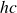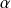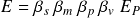# Rydberg energy

The Rydberg energy formula multiplies the Rydberg constant by the composite energy constantto find the ground state energy of an electron. The elementary form of the Rydberg constant

multiplied by the elementary form of the energy constantgives the following combined form of the equation

In elementary form, it is evident that a quantity ofincluded in both constants cancel each other, producing the correct kinetic energy operators and potential defined by the New Foundation Model

The Rydberg energy formula shows why Planck’s constant is not a fundamental constant. Both the Rydberg constant and the energy constantcontain unnecessary baggage that is removed when the two constants are multiplied together. New Foundation Model formulas use elementary Planck units in place of composite constants to show formulas in their most elementary form.

## New Foundation Model energy formula

The Rydberg energy formula shows that the fine-structure constant is the right quantity for both the momentum and velocity operators in the ground state electron.

Multiplying the Compton wavelength bygives the correct electron wavelength, and multiplying the speed of light bygives the correct velocity. The Rydberg energy formula is an application of the New Foundation Model energy formulaand can be expressed in the following form

Rydberg energy